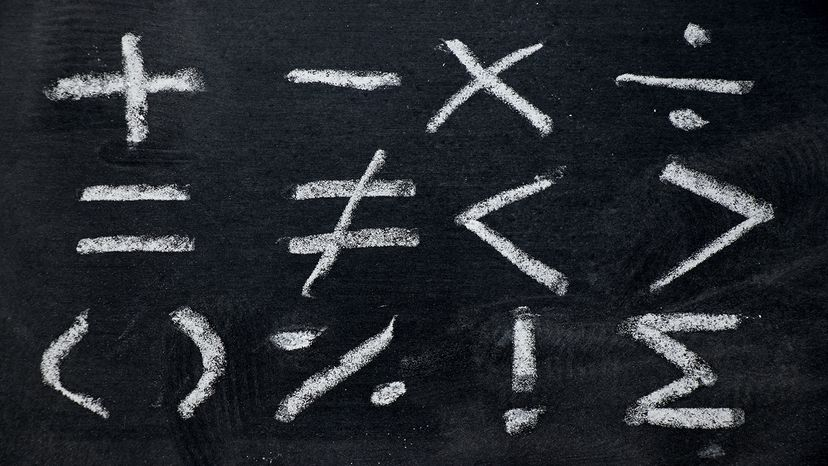# 11 Basic Math Symbols and How to Use ThemCommon mathematical symbols are the building blocks of all mathematical functions. Bankrx/Shutterstock

Common math symbols give us a language for understanding, well, everything from budgeting to the nature of reality itself. Its building blocks are relatively simple. Even the most sophisticated mathematical equations rely on a handful of fundamental common math symbols.

Before you can solve the mystery of the Collatz Conjecture, figure out a square root or understand more complex algebraic symbols, you'll need to master the basic mathematical symbols that are necessary for writing a mathematical equation.

Contents

## 1. Plus Symbol (+)

The plus symbol (+) signifies addition. It's the most basic math symbol in the world. When adding two or more numbers, use the plus symbol to indicate that you are combining them. For example, 6 + 3 means you're adding positive number 6 and positive number 3 together. You can also include the plus symbol before a number to indicate that the number is positive, although this is typically redundant — a number on its own is assumed to be positive. Writing "+3" is nonetheless a way of making clear that you're referring to positive 3.

## 2. Minus Symbol (-)

The minus symbol (-) signifies subtraction. When you're subtracting one number from another, place the minus sign between them. For instance, 6 - 3 shows that you're subtracting 3 from 6. As with the plus symbol, you can place the minus symbol in front of a number to show that it has a negative value. This is much more common, since written numbers are not negative by default. As an example, writing "-3" shows that you're referring to negative 3.

## 3. Equals Symbol (=)

The equals symbol (=) indicates that the values on either side of the symbol are not approximately equal, but are completely equivalent. In the equation 6 + 3 = 9, the equals sign indicates that the sum of 6 and 3 is equivalent to 9. The equals symbol is an essential part of any math equation.

## 4. Does Not Equal Symbol (≠)

The does not equal symbol (≠) indicates that two values are not equal. Place this sign between two numbers or mathematical expressions that are not equivalent. For example, 6 ≠ 3 states that 6 is not equal to 3.

## 5. Multiplication Symbol (×)

The multiplication symbol (×) signifies multiplying something by something else — that is, finding the product of two numbers or, to put it another way, adding a number to itself a certain number of times. Let's make that clear with an example: 6 × 3 = 18 means you're adding three 6s together, resulting in a product of 18. Since the formal multiplication symbol (×) is not common on keyboards, you can use an asterisk (*) or an "x" instead. This is particularly useful when writing computer programs or Excel formulas.

## 6. Division Symbol (÷)

The division symbol (÷) signifies the dividing of a number. This is the process of splitting a number into a certain number of equal parts. Consider the equation 6 ÷ 3 = 2. In this example, 6 divides into 3 equal groups of 2. Like one of the other key mathematical objects, the multiplication symbol, the formal symbol for division (÷) is uncommon in everyday use. When typing out equations, you can use a forward slash (/) to indicate division. Again, this is necessary for writing equations in computer programming languages.Math equations can often look like a confusing mass of random squiggles, but they are all made up of common mathematical symbols. Margarita Vin/Shutterstock

## 7. Greater Than/Less Than Symbols

The greater than symbol (>) and the less than symbol (<) don't have the same meaning, but indicate that one value is greater than another. These symbols function similarly to an equals symbol in between two numbers. For example, 6 > 3 shows that 6 is greater than 3, while 3 < 6 shows that 3 is less than 6. Remember, the larger number always faces the open end of the symbol, while the smaller number always faces the point where the two lines meet.

## 8. Greater Than or Equal To/Less Than or Equal To Symbols (≥ ≤)

The greater than or equal to symbol (≥) and the less than or equal to symbol (≤) combine the greater than and less than symbols with the equals symbol. They're used to, you guessed it, show when two values are greater (or less) than or equal to each other. This symbol is not very common in everyday usage, and it's most prevalent in equations when one or more quantities is unknown. For example, in the equation X ≥ 3, we know that X can be 3 or any number greater than 3. In this case, 3 ≥ 3 is a true statement, as is 4 ≥ 3, as is 5 ≥ 3, and so on.

## 9. Fraction Symbol (/)

The fraction symbol (/) appears as a line or slash separating two numbers, one below the other. It can appear in a few different ways. For instance, 3/5 means three-fifths. The 3 at the top of the fraction is in the position of the numerator, and the five at the bottom of the fraction is in the position of the denominator. Fractions show you how many parts of a whole you have; saying that you have 3/5 of a cookie means that if a cookie is divided into five equal parts, you have 3 of those parts. For more complicated math expressions, the fraction symbol appears as a long horizontal line separating the numerator and denominator.